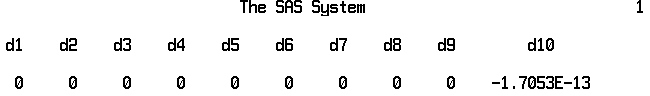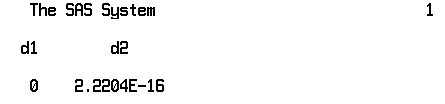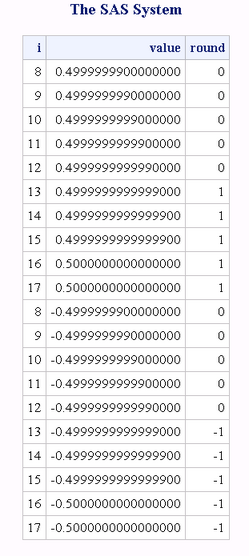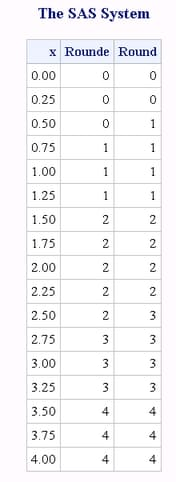# ROUND Function

Rounds the first argument to the nearest multiple of the second argument, or to the nearest integer when the second argument is omitted.

 Category: Truncation

## Syntax

ROUND (argument )

### Required Argument

#### argument

is a numeric constant, variable, or expression to be rounded.

### Optional Argument

#### rounding-unit

is a positive, numeric constant, variable, or expression that specifies the rounding unit.

## Details

### Basic Concepts

The ROUND function rounds the first argument to a value that is very close to a multiple of the second argument. The result might not be an exact multiple of the second argument.

### Differences between Binary and Decimal Arithmetic

Computers use binary arithmetic with finite precision. If you work with numbers that do not have an exact binary representation, computers often produce results that differ slightly from the results that are produced with decimal arithmetic.
For example, the decimal values 0.1 and 0.3 do not have exact binary representations. In decimal arithmetic, 3*0.1 is exactly equal to 0.3, but this equality is not true in binary arithmetic. As the following example shows, if you write these two values in SAS, they appear the same. If you compute the difference, however, you can see that the values are different.
```data _null_;
point_three=0.3;
three_times_point_one=3*0.1;
difference=point_three - three_times_point_one;
put point_three= ;
put three_times_point_one= ;
put difference= ;
run; ```
The following lines are written to the SAS log:
```   point_three= 0.3
three_times_point_one= 0.3
difference= -5.55112E-17```
Operating Environment Information: The example above was executed in a z/OS environment. If you use other operating environments, the results will be slightly different.

### The Effects of Rounding

Rounding by definition finds an exact multiple of the rounding unit that is closest to the value to be rounded. For example, 0.33 rounded to the nearest tenth equals 3*0.1 or 0.3 in decimal arithmetic. In binary arithmetic, 0.33 rounded to the nearest tenth equals 3*0.1, and not 0.3, because 0.3 is not an exact multiple of one tenth in binary arithmetic.
The ROUND function returns the value that is based on decimal arithmetic, even though this value is sometimes not the exact, mathematically correct result. In the example `ROUND(0.33,0.1)`, ROUND returns 0.3 and not 3*0.1.

### Expressing Binary Values

If the characters "0.3" appear as a constant in a SAS program, the value is computed by the standard informat as 3/10. To be consistent with the standard informat, `ROUND(0.33,0.1)` computes the result as 3/10, and the following statement produces the results that you would expect.
```if round(x,0.1) = 0.3 then
... more SAS statements ...```
However, if you use the variable Y instead of the constant 0.3, as the following statement shows, the results might be unexpected depending on how the variable Y is computed.
```if round(x,0.1) = y then
... more SAS statements ...```
If SAS reads Y as the characters "0.3" using the standard informat, the result is the same as if a constant 0.3 appeared in the IF statement. If SAS reads Y with a different informat, or if a program other than SAS reads Y, then there is no guarantee that the characters "0.3" would produce a value of exactly 3/10. Imprecision can also be caused by computation involving numbers that do not have exact binary representations, or by porting data sets from one operating environment to another that has a different floating-point representation.
If you know that Y is a decimal number with one decimal place, but are not certain that Y has exactly the same value as would be produced by the standard informat, it is better to use the following statement:
```if round(x,0.1) = round(y,0.1) then
... more SAS statements ...```

### Testing for Approximate Equality

You should not use the ROUND function as a general method to test for approximate equality. Two numbers that differ only in the least significant bit can round to different values if one number rounds down and the other number rounds up. Testing for approximate equality depends on how the numbers have been computed. If both numbers are computed to high relative precision, you could test for approximate equality by using the ABS and the MAX functions, as the following example shows.
```if abs(x-y) <= 1e-12 * max( abs(x), abs(y) ) then
... more SAS statements ...```

### Producing Expected Results

In general, `ROUND(argument, rounding-unit)` produces the result that you expect from decimal arithmetic if the result has no more than nine significant digits and any of the following conditions are true:
• The rounding unit is an integer.
• The rounding unit is a power of 10 greater than or equal to 1e-15. (If the rounding unit is less than one, ROUND treats it as a power of 10 if the reciprocal of the rounding unit differs from a power of 10 in at most the three or four least significant bits.)
• The result that you expect from decimal arithmetic has no more than four decimal places.
For example:
```options pageno=1 nodate;

data rounding;
d1 = round(1234.56789,100)     - 1200;
d2 = round(1234.56789,10)      - 1230;
d3 = round(1234.56789,1)       - 1235;
d4 = round(1234.56789,.1)      - 1234.6;
d5 = round(1234.56789,.01)     - 1234.57;
d6 = round(1234.56789,.001)    - 1234.568;
d7 = round(1234.56789,.0001)   - 1234.5679;
d8 = round(1234.56789,.00001)  - 1234.56789;
d9 = round(1234.56789,.1111)   - 1234.5432;
/* d10 has too many decimal places in the value for */
/* rounding-unit.                                   */
d10 = round(1234.56789,.11111) - 1234.54321;
run;
proc print data=rounding noobs;
run;```
Results of Rounding Based on the Value of the Rounding UnitOperating Environment Information: The example above was executed in a z/OS environment. If you use other operating environments, the results will be slightly different.

### When the Rounding Unit Is the Reciprocal of an Integer

When the rounding unit is the reciprocal of an integer, the ROUND function computes the result by dividing by the integer. (ROUND treats the rounding unit as a reciprocal of an integer if the reciprocal of the rounding unit differs from an integer in at most the three or four least significant bits.) Therefore, you can safely compare the result from ROUND with the ratio of two integers, but not with a multiple of the rounding unit. For example:
```options pageno=1 nodate;

data rounding2;
drop pi unit;
pi = arcos(-1);
unit=1/7;
d1=round(pi,unit) - 22/7;
d2=round(pi, unit) - 22*unit;
run;
proc print data=rounding2 noobs;
run;```
Results of Rounding by the Reciprocal of an IntegerOperating Environment Information: The example above was executed in an z/OS environment. If you use other operating environments, the results will be slightly different.

### Computing Results in Special Cases

The ROUND function computes the result by multiplying an integer by the rounding unit when all of the following conditions are true:
• The rounding unit is not an integer.
• The rounding unit is not a power of 10.
• The rounding unit is not the reciprocal of an integer.
• The result that you expect from decimal arithmetic has no more than four decimal places.
For example:
```data _null_;
difference=round(1234.56789,.11111) - 11111*.11111;
put difference=;
run;```
The following line is written to the SAS log:
`   difference=0`
Operating Environment Information: The example above was executed in a z/OS environment. If you use other operating environments, the results might be slightly different.

### Computing Results When the Value Is Halfway between Multiples of the Rounding Unit

When the value to be rounded is approximately halfway between two multiples of the rounding unit, the ROUND function rounds up the absolute value and restores the original sign. For example:
```data test;
do i=8 to 17;
value=0.5 - 10**(-i);
round=round(value);
output;
end;
do i=8 to 17;
value=-0.5 + 10**(-i);
round=round(value);
output;
end;
run;
proc print data=test noobs;
format value 19.16;
run;```
Results of Rounding When Values Are Halfway between Multiples of the Rounding UnitOperating Environment Information: The example above was executed in a z/OS environment. If you use other operating environments, the results might be slightly different.
The approximation is relative to the size of the value to be rounded, and is computed in a manner that is shown in the following DATA step. This DATA step code will not always produce results exactly equivalent to the ROUND function.
```data testfile;
do i = 1 to 17;
value = 0.5 - 10**(-i);
epsilon = min(1e-6, value * 1e-12);
temp = value + .5 + epsilon;
fraction = modz(temp, 1);
round = temp - fraction;
output;
end;
run;
proc print data=testfile noobs;
format value 19.16;
run;```

## Comparisons

The ROUND, ROUNDE, and ROUNDZ functions are similar with four exceptions:
• ROUND returns the multiple with the larger absolute value when the first argument is approximately halfway between the two nearest multiples of the second argument.
• ROUNDE returns an even multiple when the first argument is approximately halfway between the two nearest multiples of the second argument.
• ROUNDZ returns an even multiple when the first argument is exactly halfway between the two nearest multiples of the second argument.
• When the rounding unit is less than one and not the reciprocal of an integer, the result that is returned by ROUNDZ might not agree exactly with the result from decimal arithmetic. ROUND and ROUNDE perform extra computations, called fuzzing, to try to make the result agree with decimal arithmetic in the most common situations. ROUNDZ does not fuzz the result.

## Example

The following example compares the results that are returned by the ROUND function with the results that are returned by the ROUNDE function. The output was generated from the UNIX operating environment.
```data results;
do x=0 to 4 by .25;
Rounde=rounde(x);
Round=round(x);
output;
end;
run;
proc print data=results noobs;
run;```
Results That Are Returned by the ROUND and ROUNDE Functions